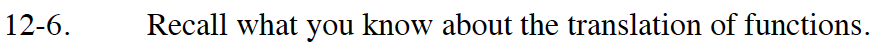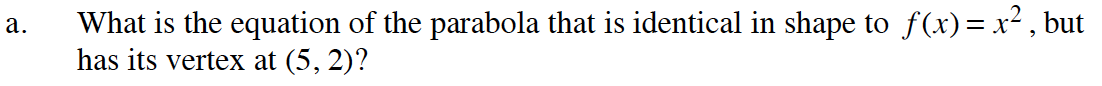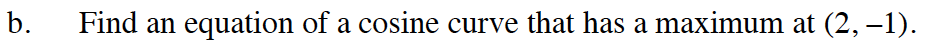### Home > CALC > Chapter Ch12 > Lesson 12.1.1 > Problem12-6

12-6.Recall that vertex form for a parabola is y = a(xh)2 + k.The function f(x) = cos(x) has a maximum point at (0, 1).
How far does the original function need to be translated horizontally? Vertically?